# Navigation and Chart work - Working out the course to steer

Before we make a passage across a current we must consider if we needto steer a course to allow for the tide. If there is a tide running across the route and instead of allowing for it, we just point the boat to the destination, we will end up sailing in an arc. This will take longer than doing some quick calculations first.

Not only will this approach take longer, but also you may be swept into a angerous area or in a sailing boat, you may end up head to wind and unable to sail.

The first step is to draw in the ground track to the destination.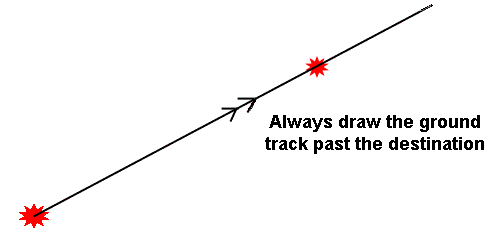When you do this, you must draw the line past the point where you intend to stop, even if the line goes over the land! If you do not do this, sometimes you will be unable to complete the diagram correctly.

The next step is to measure the distance from the start to the destination, and by comparing this to the speed you expect to make decide roughly how long the passage will take.

When motoring the speed is easy to deduce but if you are sailing it is not so easy. With practice you will learn to guess what the speed will be in your boat on any heading. During the course you will always be told the boat's speed.

When we work out the approximate time of passage we are only looking to see if it will be 1 hour or more so that we know how much tidal information to look up.

E.g.

• A passage of 6M at 5kn will take about 1 hour.
• A passage of 11M at 6kn will take about 2 hours.
• A passage of 5M at 10kn will take half an hour.

These figures take no account of the tide, so there is no point in trying to be very accurate at this stage. We will know the start time of the passage, so if it is going to take about 1 hour, we need to find the tidal stream information for that hour. If we expect it to take two hours, we need to find the tidal stream for those 2 hours.

We do this in exactly the same manner as for working out an EP. The only difference is we are not given the start and finish time; we need to work out the finish time for ourselves. This is easy to do.

A passage of 1 hour starting at 1024 will need tidal information for the period from 1024 to 1124.

A passage of 3 hours starting at 1345 will need tidal information for the period from 1345 to 1645.

We then need to plot the tidal vector, from our start point!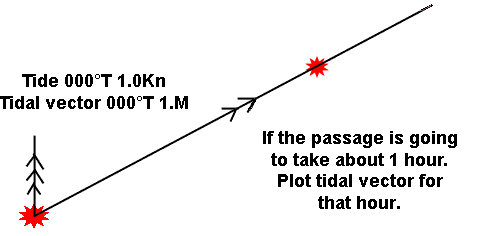For us to work out the course to steer we must draw a triangle, each side of which represents the same period of time. So our next step is to measure off the distance we expect the boat to travel through the water in 1 hour. In this case 5M. This distance is measured from the end of the tidal vector on to the ground track.If we then draw this line in, it becomes the water track. It does not matter that the water track has not reached the destination, because you can assume that for a short period, conditions will remain similar, and you can continue on the same course. In practice, you would probably be able to see the destination and could approach it by eye.The other possibility is that the water track will reach the ground track after the destination. When this happens, it means that the passage will take less than 1 hour, when you arrive at the destination you will stop!

This is the reason that we always draw the ground track past the destination, even if it passes over land. If you have not done this, you will almost certainly plot the water track to the destination.

The water track will very rarely arrive exactly at the destination, if it does when you are doing any training exercises you should check your workings carefully as this is unlikely to happen.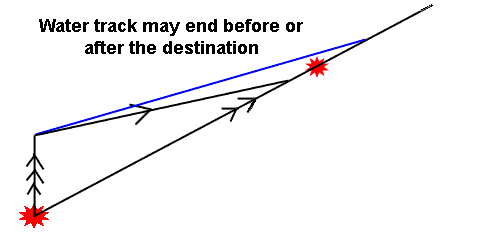The reason it is incorrect is that each side of the triangle must represent the same period of time. If the tidal vector represents the distance the tide will travel in one hour, the water track must also.

Because of this you may have a course to steer which ends up running over the land, provided this is after the point you are aiming for it is not a problem. The course to steer line very rarely ends at the destination, if it does, check through the process again. Make sure you have measured off the distance you expect the boat to travel through the water, from the end of the tide vector!

Question 1. Chart 3. Variation = 7°W.

The position of the yacht by GPS is 45°42.8'N 06°18.37'W. What is the course to steer to reach the Quaker Safe Water buoy (45°40.7'N 06°13.81'W), if the boat's speed is 3.9Kn and the tide is 180°T at 1.5Kn?

Question 2. Chart 3. Variation = 7°W.

Starting from 45°54.0'N06°00.0'W, what is the course to steer to reach the leading line at 45°51.8'N 05°49.1'W, if the boat's speed is 8.1Kn and the tide is 350°T at 1.9Kn?

##### Working out the time of passage

The formula for working out how long a passage will take is very simple.

The time = The distance to travel / the speed of the vessel in that direction.

T = D/S

The tricky part is deciding on the vessel's speed. It is not the speed given in the question, that is the speed through the water and takes no account of the tidal effect.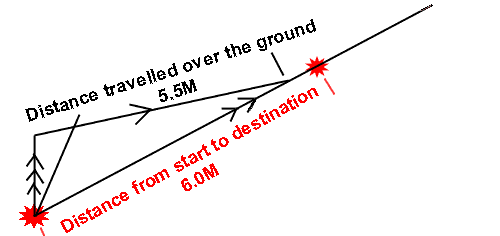To find the speed over the ground (SOG) we go back to the diagram and measure the distance from the start point to the point where the water track meets the ground track. If this distance is 5.5M and we have constructed the diagram for one hour, the vessels SOG is 5.5Kn.

If the diagram had been based on a half hour passage the SOG would be 11.0Kn because the vessel would cover 5.5M in half an hour.

The calculation is as follows:

T = D/S

T = 6.0M/5.5Kn

T = 1.09 hours

If we multiply this by 60 minutes we have our time of passage in minutes.

T = 1.09 X 60 = 65 minutes.

Question 3.

In the previous two questions, calculate how long the passage will take.

Question 4. Chart 3. Variation = 7°W.

The bearing and distance to the waypoint at the centre of the compass rose west of Slade Island is 170°T 12.4M.

A) From this position what is the course to steer to reach the Synka Buoy (46°10.57'N 05°53.37'W) if the vessel's speed is 3.6kn and the tide is 016°T at 2.0kn?

B) What is the vessel's speed over the ground (SOG)?

C) How long will the passage take?

To answer the next questions you will need to calculate the correct tidal information yourself. The information is in theTraining Almanac, use the table for Victoria.

Question 5.

On 4 th October, at 2213 Summer Time and starting from 45°43.0'N 05°40.0'W.

A) What is the course to steer to reach the Safe Water Buoy (45°40.8'N 05°48.4'W), if the boat's speed is expected to be 6.9Kn?

B) What will be the ETA ? Use Diamond Q.

##### Passages shorter than 1 hour

When you measure the distance to the destination and compare it to the expected speed of the vessel you may decide that the passage will take much less than one hour. If there is sufficient space on the chart you can still create the diagram for one hour, however it is likely that the diagram may be too large to fit on the chart.

In this case you can use any time period that is suitable. You may choose to draw the diagram for 20 or 30 minutes. The only rule is that each side of the diagram must be for the same period of time.

If the tide is 090°T at 2.0Kn, the tidal vector is the diagram is drawn for half an hour should be 090°T 1.0M, equally if the vessel's speed is 6.0Kn, the water track should be 3.0M long, as this is the distance it will travel in 30 minutes.

Question 6.

On 24th May a vessel is in position 45°57.7'N 05°40.00'W at 1346 Summer Time making 9.0kn. Use Diamond K.

A) What is the course to steer for a point 1.2M north of the Christopher Point Light House?

B) What will be the ETA ?

##### Passage of more than 1 hour

If the passage will take longer than one hour and it is in open water, the best approach is to find all the tidal information for the period of the passage, then plot the all the tidal vectors first followed by one long water track.The other approach is to work out two separate courses to steer, one for each hour. This will work, but the passage will take longer and the navigation will involve more work.

The passage will take longer because some portion of the tides often cancels out, the longer the time of passage the more this will happen. If a cross channel trip from France to the the South Coast of England takes 12 hours, it is likely that most of the tides will be cancelled out as they will flow to the east for 6 hours then back to the west for 6 hours.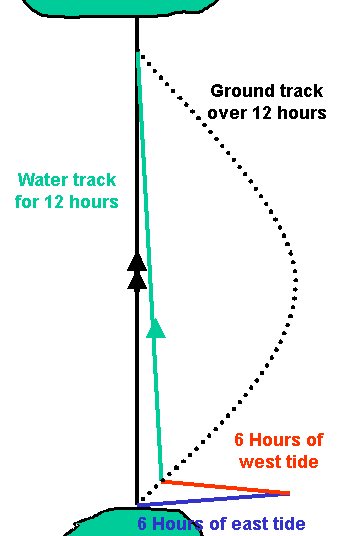Question 7. Chart 3. Variation = 7°W.

On 25th April, at 2244 Summer Time a vessel is in position 45°43.0'N 06°20.0'W. Use Diamond M.

A) What is the course to steer to 45°52.0'N 06°20.0'W if the predicted boat speed is 4.5Kn?

B) What is the ETA?

##### Leeway with a course to steer

If there is leeway when you are working out the course to steer there is no change in the calculations. Everything is worked out as if there was no leeway, but the last step once you have the water track is to decide if the leeway is to be added or subtracted from the water track to find the correct course to steer. This is the course that is passed to the helmsman.

Leeway is applied in to the wind with a course to steer as you are countering the effect it is going to have.

Question 8. Use Chart RYA4 F.

On March 22nd in thick fog, a vessel is in position 46°17.2'N 05°57.30'W at 1326UT. Use Diamond C.

A) What is the course to steer to reach the N Potta Buoy (46°16.2'N 05°59.03'W) if the boat speed is 4.6Kn and the leeway in a northerly wind of 10°?

B) What is the ETA ?

Finding the compass course to steer.

When a question asks for the "Compass course" you need to apply deviation from the deviation table on the back of the Training Almanac.

Do all the previous calculations including dealing with leeway if there is any, then apply the deviation as the last step. This is because the leeway may be large enough to change the figure for deviation used from the table.

Question 9. Chart 4F. Use the deviation table at the back of the Training Almanac.

On the 7th October at 1020 Summer Time, a vessel starts a passage from the Range Head buoy (46°15.47'N 05°52.07'W) to the NE Groats Buoy (46°14.04'N 05°51.5'W). If the vessel's speed is 3.2kn and the leeway from a westerly wind is 10°;

A) What is the compass course to steer?

B) What is the ETA ? Use Diamond E.

Non spring or neap tides.

At the Coastal Skipper level the questions used will not be neat spring tide or neap tide days, the tides are likely to be something other than the standard figures. In these cases you should use the computation of rates table on page 26 of the Training Almanac to calculate the correct tidal rate for that day.

Question 10. Chart 3. Use the deviation table at the back of the Training Almanac and the Computation of Rates table on page 26.

On the 19th May at 1505 Summer Time a vessel is in position 46°00.0'N 05°50.0'W. If its speed is 15Kn and the expected leeway in a southerly wind is 5°?

A) What is the compass course to reach 45°59.0'N 006°00.00'W?

B) What is the ETA?